Search by Topic

Resources tagged with PrimaryResourceful similar to Baravelle:

Filter by: Content type:
Age range:
Challenge level:

There are 28 results

Broad Topics > Habits of Mind > PrimaryResourcefulBaravelle

Age 7 to 16 Challenge Level:

What can you see? What do you notice? What questions can you ask?Eight Hidden Squares

Age 7 to 14 Challenge Level:

On the graph there are 28 marked points. These points all mark the vertices (corners) of eight hidden squares. Can you find the eight hidden squares?Satisfying Four Statements

Age 7 to 11 Challenge Level:

Can you find any two-digit numbers that satisfy all of these statements?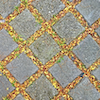Seeing Squares

Age 5 to 11 Challenge Level:

Players take it in turns to choose a dot on the grid. The winner is the first to have four dots that can be joined to form a square.Cops and Robbers

Age 7 to 16 Challenge Level:

Can you find a reliable strategy for choosing coordinates that will locate the robber in the minimum number of guesses?Poly Plug Rectangles

Age 5 to 14 Challenge Level:

The computer has made a rectangle and will tell you the number of spots it uses in total. Can you find out where the rectangle is?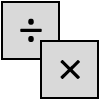Four Go

Age 7 to 11 Challenge Level:

This challenge is a game for two players. Choose two of the numbers to multiply or divide, then mark your answer on the number line. Can you get four in a row?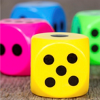Dicey Operations in Line

Age 7 to 11 Challenge Level:

Who said that adding, subtracting, multiplying and dividing couldn't be fun?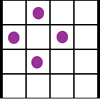Square Corners

Age 7 to 11 Challenge Level:

What is the greatest number of counters you can place on the grid below without four of them lying at the corners of a square?First Connect Three

Age 7 to 14 Challenge Level:

The idea of this game is to add or subtract the two numbers on the dice and cover the result on the grid, trying to get a line of three. Are there some numbers that are good to aim for?Play to 37

Age 7 to 11 Challenge Level:

In this game for two players, the idea is to take it in turns to choose 1, 3, 5 or 7. The winner is the first to make the total 37.Sponge Sections

Age 7 to 11 Challenge Level:

You have been given three shapes made out of sponge: a sphere, a cylinder and a cone. Your challenge is to find out how to cut them to make different shapes for printing.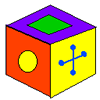A Puzzling Cube

Age 7 to 11 Challenge Level:

Here are the six faces of a cube - in no particular order. Here are three views of the cube. Can you deduce where the faces are in relation to each other and record them on the net of this cube?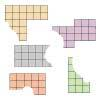Torn Shapes

Age 7 to 11 Challenge Level:

These rectangles have been torn. How many squares did each one have inside it before it was ripped?Cows and Sheep

Age 7 to 11 Challenge Level:

Use your logical reasoning to work out how many cows and how many sheep there are in each field.Coded Hundred Square

Age 7 to 11 Challenge Level:

This 100 square jigsaw is written in code. It starts with 1 and ends with 100. Can you build it up?Sealed Solution

Age 7 to 11 Challenge Level:

Ten cards are put into five envelopes so that there are two cards in each envelope. The sum of the numbers inside it is written on each envelope. What numbers could be inside the envelopes?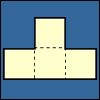Cut Nets

Age 7 to 11 Challenge Level:

Each of the nets of nine solid shapes has been cut into two pieces. Can you see which pieces go together?Nice or Nasty

Age 7 to 14 Challenge Level:

There are nasty versions of this dice game but we'll start with the nice ones...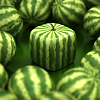Shape Times Shape

Age 7 to 11 Challenge Level:

These eleven shapes each stand for a different number. Can you use the number sentences to work out what they are?Mystery Matrix

Age 7 to 11 Challenge Level:

Can you fill in this table square? The numbers 2 -12 were used to generate it with just one number used twice.The Remainders Game

Age 7 to 14 Challenge Level:

Play this game and see if you can figure out the computer's chosen number.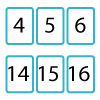Twenty Divided Into Six

Age 7 to 11 Challenge Level:

Katie had a pack of 20 cards numbered from 1 to 20. She arranged the cards into 6 unequal piles where each pile added to the same total. What was the total and how could this be done?Number Lines in Disguise

Age 7 to 14 Challenge Level:

Some of the numbers have fallen off Becky's number line. Can you figure out what they were?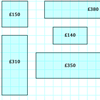Through the Window

Age 7 to 11 Challenge Level:

My local DIY shop calculates the price of its windows according to the area of glass and the length of frame used. Can you work out how they arrived at these prices?Magic Vs

Age 7 to 11 Challenge Level:

Can you put the numbers 1-5 in the V shape so that both 'arms' have the same total?Dotty Six

Age 5 to 11 Challenge Level:

Dotty Six is a simple dice game that you can adapt in many ways.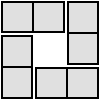4 Dom

Age 5 to 16 Challenge Level:

Use these four dominoes to make a square that has the same number of dots on each side.